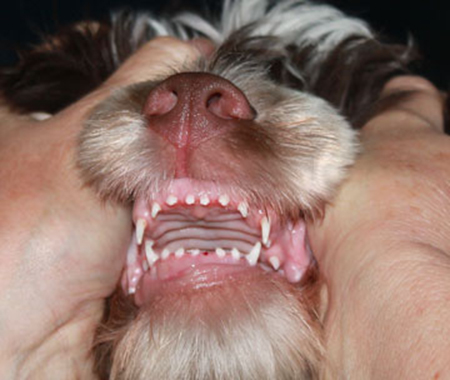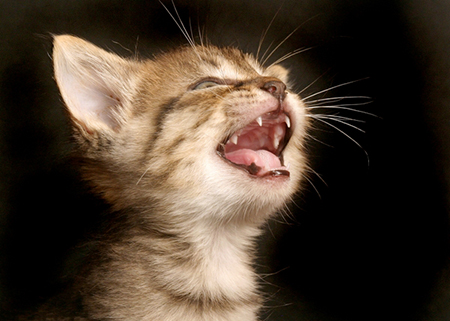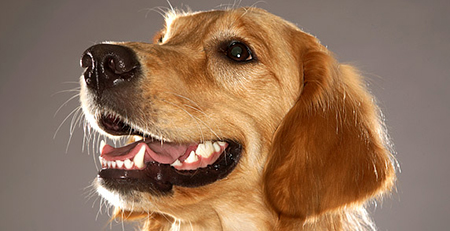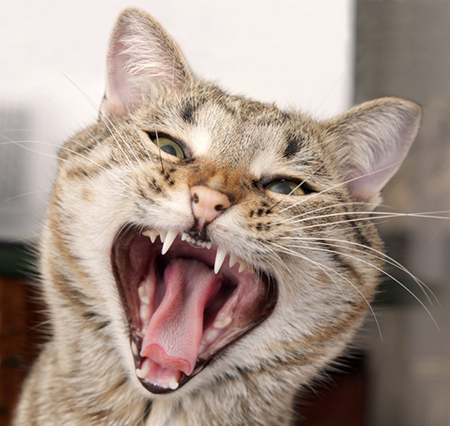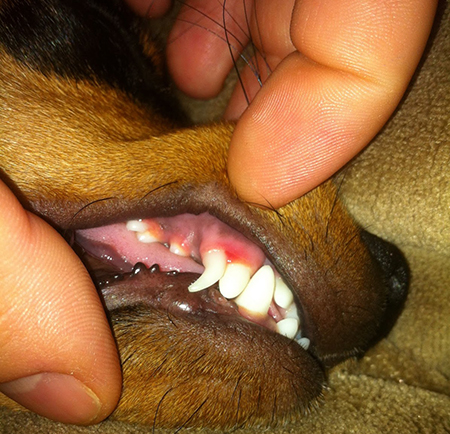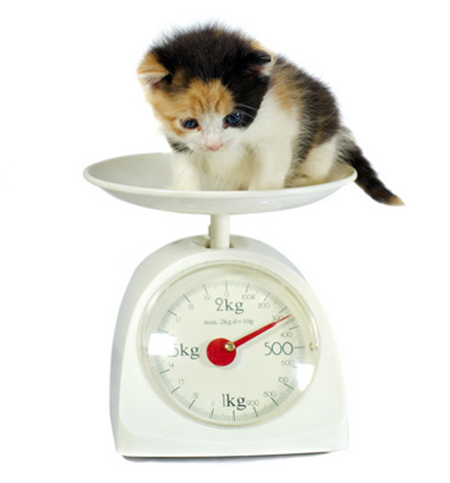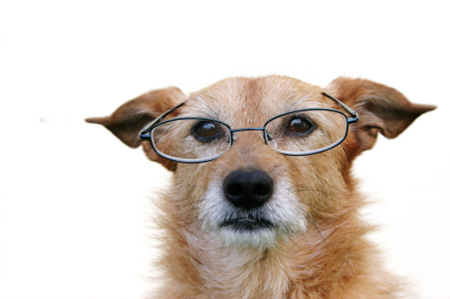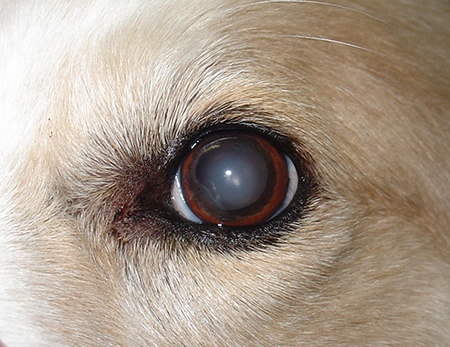From: 萌爪医生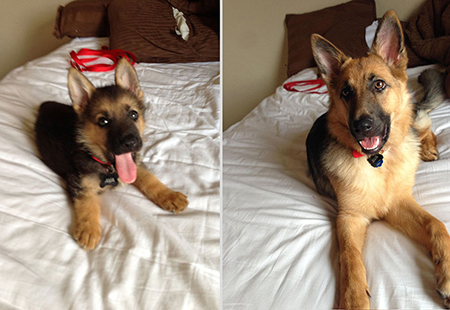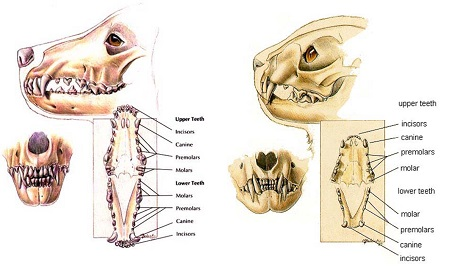I = Incisor = 门牙；Di = Deciduous Incisor = 乳牙门牙
C = Canine = 犬；; Dc = Deciduous Canine = 乳牙犬牙
P = Premolar = 前臼齿； Dp = Deciduous Premolar = 乳牙前臼齿
M = Molar = 臼齿